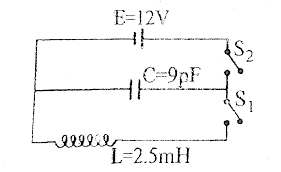In the circuit shown, the capacitor is initially charged with a 12V battery, when switch  S1 is open and switch S2 is closed. S1   is then closed and, at the same time, S2 is opened. The maximum value of current in the circuit is

# In the circuit shown, the capacitor is initially charged with a 12V battery, when switch  ${S}_{1}$ is open and switch ${S}_{2}$ is closed. ${S}_{1}$   is then closed and, at the same time, ${S}_{2}$ is opened. The maximum value of current in the circuit is1. A

0.72mA

2. B

0.84mA

3. C

0.38mA

4. D

0.1mA.

Fill Out the Form for Expert Academic Guidance!l

+91

Live ClassesBooksTest SeriesSelf Learning

Verify OTP Code (required)

### Solution:

$\begin{array}{l}\frac{1}{2}L{i}^{2}=\frac{1}{2}C{v}^{2}\\ i=v\sqrt{\frac{C}{L}}=12\sqrt{\frac{9X{10}^{-12}}{2.5X{10}^{-3}}}=\frac{12X3}{5}\sqrt{{10}^{-8}}i=7.2X{10}^{-4}A\end{array}$

## Related content

 Differences & Comparisons Articles in Physics Important Topic of Physics: Reynolds Number Distance Speed Time Formula Refractive Index Formula Mass Formula Electric Current Formula Electric Power Formula Resistivity Formula Weight Formula Linear Momentum Formula+91

Live ClassesBooksTest SeriesSelf Learning

Verify OTP Code (required)